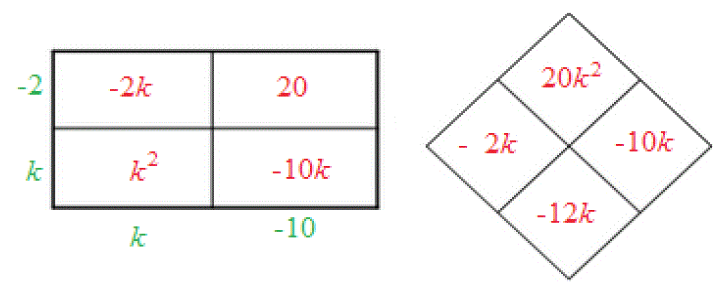### Home > AC > Chapter 8 > Lesson 8.1.3 > Problem8-29

8-29.

Factor the following quadratics, if possible.

1. $k^2-12k+20$

Use a generic rectangle and a diamond to solve.1. $6x^2+17x-14$

$\left(2x+7\right)\left(3x-2\right)$

1. $x^2-8x+16$

1. $9m^2-1$

Insert a $0m$ between the $9m^2$ and the $-1$.